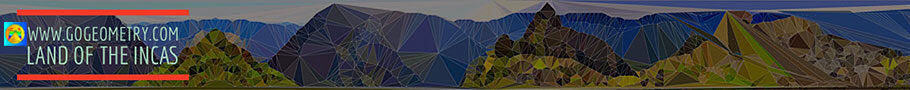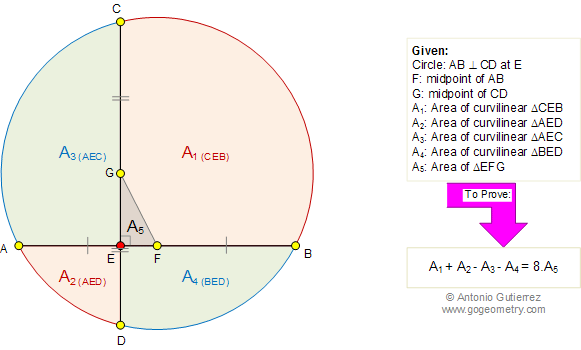# Problem 578: Circle, Perpendicular Chords, Midpoints, Curvilinear Triangle, Area, 90 Degrees. Level: Math Education, High School, SAT Prep, College

 The figure shows a circle with a chord AB perpendicular to chord a CD at E. F is the midpoint of AB and G is the midpoint of CD. A1, A2, A3, and A4, are the areas of curvilinear triangles CEB, AEC, AED, and BED, respectively. If A5 is the area of triangle EFG, prove that A1+ A2 - A3 - A4 = 8.A5 .Home | Search | Geometry | Problems | All Problems | Visual Index | 571-580 | Areas | Perpendicular Chords | Email | Solution / comment | By Antonio Gutierrez